# RD Sharma Solutions Ex-12.1, Heron's Formula, Class 9, Maths Class 9 Notes | EduRev

## Class 9 : RD Sharma Solutions Ex-12.1, Heron's Formula, Class 9, Maths Class 9 Notes | EduRev

The document RD Sharma Solutions Ex-12.1, Heron's Formula, Class 9, Maths Class 9 Notes | EduRev is a part of the Class 9 Course RD Sharma Solutions for Class 9 Mathematics.
All you need of Class 9 at this link: Class 9

Q1 . Find  the area of a triangle whose sides are respectively 150 cm, 120 cm and 200 cm.

Solution:

Let the sides of the given triangle be a, b, c respectively.

So given,

a = 150 cm

b = 120 cm

c = 200 cm

By using Heron’s Formula

The area of the triangle =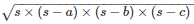Semi perimeter of a triangle = s

2s = a + b + c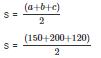s = 235 cm

Therefore, area of the triangle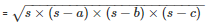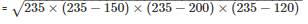= 8966.56cm2

Q2. Find  the area of a triangle whose sides are respectively 9 cm, 12 cm and 15 cm.

Solution:

Let the sides of the given triangle be a, b, c respectively.

So given,

a = 9 cm

b = 12 cm

c = 15 cm

By using Heron’s Formula

The area of the triangle =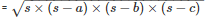Semi perimeter of a triangle = s

2s = a + b + c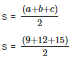s = 18 cm

Therefore, area of the triangle   =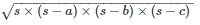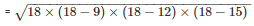= 54cm2

Q3. Find the area of a triangle two sides of which are 18 cm and 10 cm and the perimeter is 42cm.

Solution:

Whenever we are given the measurements of all sides of a triangle, we basically look for Heron’s formula to find out the area of the triangle.

If we denote area of the triangle by A, then the area of a triangle having sides a, b, c and s as semi-perimeter is given by: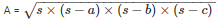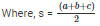We are given:

a = 18 cm

b = 10 cm, and perimeter = 42 cm

We know that perimeter = 2s,

So, 2s = 42

Therefore, s = 21 cm

We know that,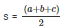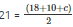42 = 28 + c

c = 14 cm

So the area of the triangle is: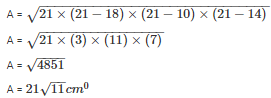Q4 . In a triangle ABC, AB = 15cm, BC = 13cm and AC = 14cm. Find the area of triangle ABC and hence its altitude on AC.

Solution:

Let the sides of the given triangle be AB = a, BC = b, AC =  c respectively.

So given,

a = 15 cm

b = 13 cm

c = 14 cm

By using Heron’s Formula

The area of the triangle  =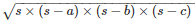Semi perimeter of a triangle= 2s

2s = a + b + c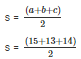s = 21 cm

Therefore, area of the triangle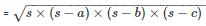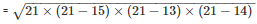= 84cm2

BE is a perpendicular on AC

Now, area of triangle = 84cm2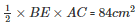BE = 12cm

The length of BE is 12 cm

Q5 . The perimeter of a triangular field is 540 m and its sides are in the ratio 25:17:12. Find the area of triangle.

Solution:

Let the sides of the given triangle be a = 25x, b = 17x, c = 12x respectively,

So,

a = 25x cm

b = 17x cm

c = 12x cm

Given Perimeter = 540 cm

2s = a + b + c

a + b + c = 540 cm

25x + 17x + 12x = 540 cm

54x = 540 cm

x = 10 cm

Therefore, the sides of a triangle are

a = 250 cm

b = 170 cm

c = 120 cm

Now, Semi perimeter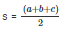= 540/2

= 270 cm

By using Heron’s Formula

The area of the triangle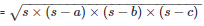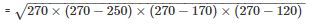= 9000cm2

Therefore, the area of the triangle is 9000cm2

Q6. The perimeter of a triangle is 300 m. If its sides are in the ratio of 3: 5: 7. Find the area of the triangle.

Solution:

Given the perimeter of a triangle is 300 m and the sides are in a ratio of 3: 5: 7

Let the sides a, b, c of a triangle be 3x, 5x, 7x respectively

So, the perimeter = 2s = a + b + c

200 = a + b + c

300 = 3x + 5x + 7x

300 = 15x

Therefore, x = 20 m

So, the respective sides are

a = 60 m

b = 100 m

c = 140 m

Now, semi perimeter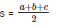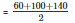= 150 m

By using Heron’s Formula

The area of a triangle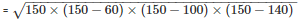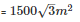Thus, the area of a triangle is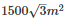Q7. The perimeter of a triangular field is 240 dm. If two of its sides are 78 dm and 50 dm, find the length of the perpendicular on the side of length 50 dm from the opposite vertex.

Solution:

Given,

In a triangle ABC, a = 78 dm = AB, b = 50 dm = BC

Now, Perimeter = 240 dm

Then, AB + BC + AC = 240 dm

78 + 50 + AC = 240

AC = 240-(78+50)

AC = 112 dm = c

Now, 2s = a + b + c

2s = 78 + 50 + 112

s = 120 dm

Area of a triangle ABC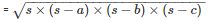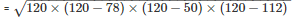= 1680dm2

Let AD be a perpendicular on BC

Area of the triangle ABC =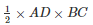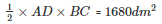AD = 67.2 dm

Q8. A triangle has sides 35 cm, 54 cm, 61 cm long. Find its area. Also, find the smallest of its altitudes?

Solution:

Given,

The sides of the triangle are

a = 35 cm

b = 54 cm

c = 61 cm

Perimeter 2s = a + b + c

2s = 35 + 54 + 61 cm

Semi perimeter s = 75 cm

By using Heron’s Formula,

Area of the triangle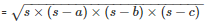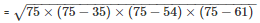= 939.14cm2

The altitude will be smallest provided the side corresponding to this altitude is longest.

The longest side = 61 cm

Area of the triangle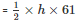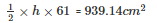h = 30.79cm

Hence the length of the smallest altitude is 30.79cm

Q9.The lengths of the sides of a triangle are in a ratio of 3 : 4 : 5 and its perimeter is 144 cm. Find the area of the triangle and the height corresponding to the longest side?

Solution:

Given the perimeter of a triangle is 160m and the sides are in a ratio of  3 : 4 : 5

Let the sides a, b, c of a triangle be 3x, 4x, 5x respectively

So, the perimeter = 2s = a + b + c

144 = a + b + c

144 = 3x+ 4x+ 5x

Therefore, x = 12cm

So, the respective sides are

a = 36cm

b = 48cm

c = 60cm

Now, semi perimeter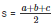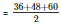= 72 cm

By using Heron’s Formula

The area of a triangle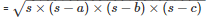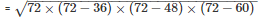= 864cm2

Thus, the area of a triangle is 864cm2

The altitude will be smallest provided the side corresponding to this altitude is longest.

The longest side = 60 cm

Area of the triangle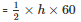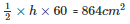h = 28.8 cm

Hence the length of the smallest altitude is 28.8 cm

Q10. The perimeter of an isosceles triangle is 42 cm and its base is 3/2 times each of the equal side. Find the length of each of the triangle, area of the triangle and the height of the triangle.

Solution:

Let ‘x’ be the length of two equal sides,

Therefore the base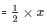Let the sides a, b, c of a triangle be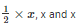respectively

So, the perimeter = 2s = a + b + c

42 = a + b + c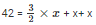Therefore, x = 12 cm

So, the respective sides are

a = 12 cm

b = 12 cm

c = 18 cm

Now, semi perimeter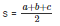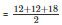= 21 cm

By using Heron’s Formula

The area of a triangle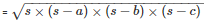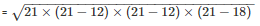= 71.42cm2

Thus, the area of a triangle is 71.42cm2

The altitude will be smallest provided the side corresponding to this altitude is longest.

The longest side = 18 cm

Area of the triangle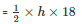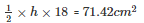h = 7.93 cm

Hence the length of the smallest altitude is 7.93 cm

Q11. Find the area of the shaded region in fig. below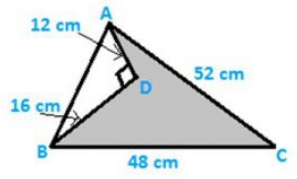Solution:

Area of the shaded region = Area of ΔABC−Area of ΔADB

Now in triangle ADB

AB2 = AD2+BD2………(i)

Given, AD = 12 cm, BD =16 cm

Substituting the value of AD and BD in eq (i), we get

AB2 = 122+162

= 400cm2

AB = 20 cm

Now, area of a triangle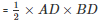= 96cm2

Now in triangle ABC,

The area of a triangle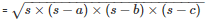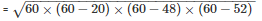= 480cm2

Thus, the area of a triangle is 480cm2

Area of shaded region = Area of triangle ABC – Area of triangle ADB

= (480 – 96) cm2

= 384 cm2

Area of shaded region = 384 cm2

Offer running on EduRev: Apply code STAYHOME200 to get INR 200 off on our premium plan EduRev Infinity!

91 docs

,

,

,

,

,

,

,

,

,

,

,

,

,

,

,

,

,

,

,

,

,

,

,

,

,

,

,

,

,

,

;